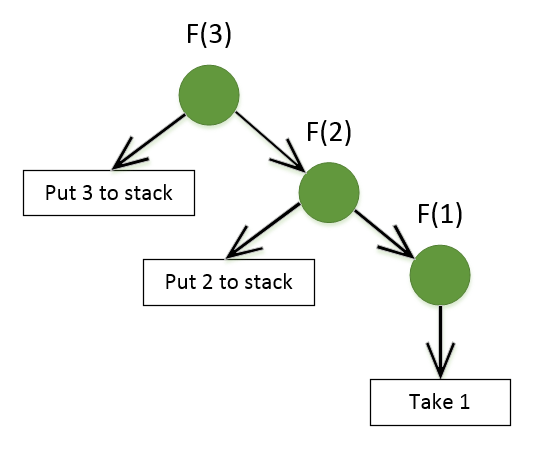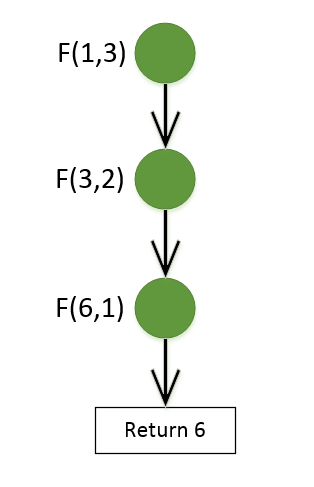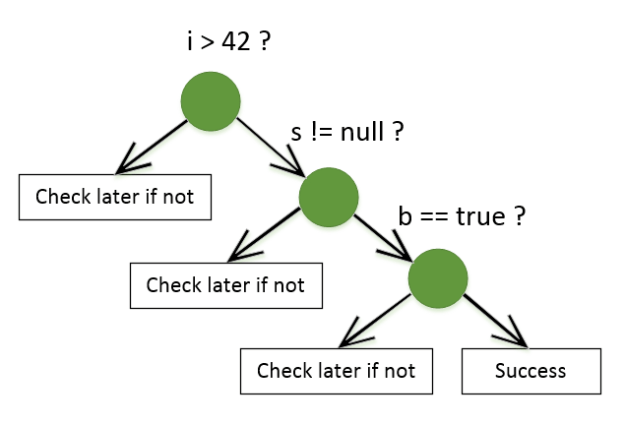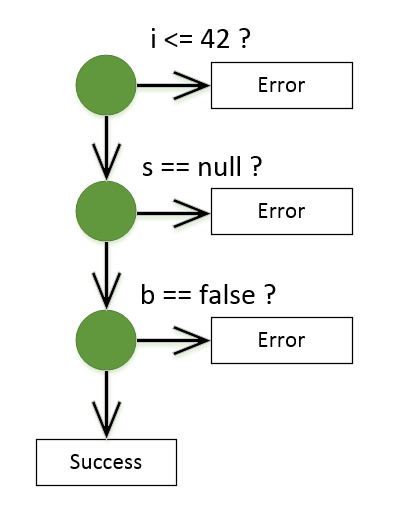# Early exit is a tail call optimization of procedural languages

A couple of days ago, a nice analogy came to mind which I thought would be an interesting one to share: early exit is essentially a tail call optimization of procedural languages. Let’s see how it is so.

## Tail call optimization

Before explaining the metaphor, let me give you a refresher for what a tail call and the tail call optimization are.

A tail call is basically any call that is performed last in a method. Let’s look at a simple example of it:

``````public int Run(int integer)
{
Log(integer);
return Calculate(integer); // Tail call
}

private int Calculate(int integer)
{
return integer + 1;
}
``````

The Calculate method invocation here is a tail call because it is executed at the end of the Run method. Now, compare it to the following code:

``````public int Run(int integer)
{
Log(integer);
return 2 + Calculate(integer); // NOT a tail call
}

private int Calculate(int integer)
{
return integer + 1;
}
``````

In this example, the Calculate method invocation is not the last one: Run still needs to perform the “plus 2” operation after it gets the result from Calculate. Therefore, it is not a tail call.

The tail call optimization eliminates the necessity to add a new frame to the call stack while executing the tail call. For the first code sample, such optimization would have the same effect as inlining the Calculate method (although compiler doesn’t perform the actual inlining, it gives CLR a special instruction to perform a tail call optimization during JIT-compilation):

``````public int Run(int integer)
{
Log(integer);
return integer + 1; // Tail call optimization
}
``````

Such optimization is not guaranteed in C# and that’s basically fine because there are few cases where a typical C# application would benefit from it anyway.

The place where the tail call optimization is essential is functional languages, which highly rely on recursion. Without it, long-running recursive functions would exhaust the call stack and fail due to stack overflow exceptions.

A tail call in a recursive function has a special name: tail recursion. There’s also a special kind of optimization for it in functional languages: they guarantee that a properly tail-recursive implementation would be converted to a loop.

## Recursive call optimization

Let’s look at the following function:

``````// Version without proper tail recursion
let rec factorial n =
match n with
| 0 | 1 -> 1
| _ -> n * factorial (n - 1)

factorial 3 // returns 6``````

It’s a recursive function that calculates factorial of a number. Note that the compiler can’t apply the optimization here because this implementation is not properly tail-recursive.

When we try to execute it with 3 as a parameter, it goes like this:Recursion without tail call optimization

With every recursive call, the program has to memorize its current location in order to be able to eventually get back and multiply all values with each other. Obviously, such implementation is limited by the size of the stack as we put additional pressure on it with every call.

To get the benefits of tail call optimization, we can refactor the function introducing an additional accumulator parameter:

``````// Version with a proper tail recursion
let rec factorial acc n =
match n with
| 0 | 1 -> acc
| _ -> factorial (acc * n) (n - 1)

factorial 1 3 // returns 6``````

This time, the compiler is able to avoid the use of additional stack space while calling the function and effectively transforms it into a loop:Recursion with tail call optimization

As you can see, this implementation doesn’t use the stack to memorize the previous values, it just passes an intermediate result along with the next value of N.

## Early exit

So, how is this related to early exit? Early exit is a refactoring technique that helps reduce indentation and thus simplify the code. Let’s look at an example:

``````public string Execute(int integer, string str, bool boolean)
{
string result;

if (integer > 42)
{
if (!string.IsNullOrWhiteSpace(str))
{
if (boolean)
{
result =  "Success";
}
else
{
result = "Error: Incorrect boolean";
}
}
else
{
result = "Error: String is null or empty";
}
}
else
{
result = "Error: Integer is too small";
}

return result;
}
``````

When you read such code, you need to go through its branches and make sure you don’t forget to examine all of them:Code without Early Exit refactoring

Each time you go one `if` deeper, you have to memorize the `else` branch in order to explore it later. This code exhausts your mental stack. That’s why the early exit refactoring is so effective. It helps you “flatten” the reading pattern and alleviate the burden on your brain.

The code above can be transformed into the following one:

``````public string Execute(int integer, string str, bool boolean)
{
if (integer <= 42)
return "Error: Integer is too small";

if (string.IsNullOrWhiteSpace(str))
return "Error: String is null or empty";

if (!boolean)
return "Error: Incorrect boolean";

return "Success";
}
``````

Which takes us much less effort to understand:Code with Early Exit refactoring

Just as the recursive call optimization removes the limit imposed by the call stack size, the early exit technique takes off the limitation imposed by our mental stack.

## Conclusions

No conclusions here, actually, just hope you found this analogy interesting. It followed me for several days and I felt like I had to post it here :)

## Subscribe

I don't post everything on my blog. Don't miss smaller tips and updates. Sign up to my mailing list below.